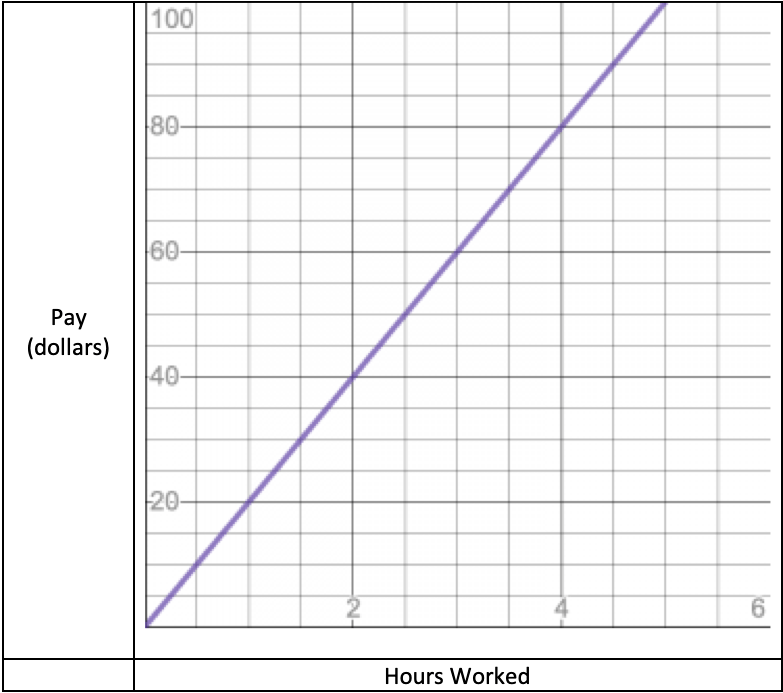## Comparing Data in Different Formats

This post about comparing data in different formats is part of a series of posts to help you prepare for the Advanced Algebra and Functions part of the Accuplacer test.

## QuestionLiam’s Pay

This graph shows how much Liam gets paid as a function of the number of hours he works. Sarah, on the other hand, is paid according to the formula S=(75/4)h, where S represents Sarah’s pay and h represents the number of hours she has worked.

Is Liam’s rate of pay more or less than Sarah’s, and by how much?

## Solution

You’re being asked to compare data that measure similar things but are given to you in different formats.

The trick is to convert one of those expressions into the same format as the other. Or, if you can’t do that, then convert both to some third format.

P=(75/4)h looks . . . almost straightforward. That fraction may be inconvenient. 75/4 = 18.75, so let’s say S = 18.75h represents Sarah’s rate of pay.

Now look at the graph. Can you represent Liam’s pay as something like L=kh, where k is Liam’s rate of pay? Since the graph goes through the origin, its equation will be of the form L=something*and “something” is the slope. To find it from the graph, pick two points on the line that are easy to read, and calculate. The origin — with coordinates (0, 0) — looks easy. And the point (2, 40) doesn’t look bad. The slope is the change in y divided by the change in for those two points:

So Liam’s pay is L=20h.

You’re asked for the difference between Liam’s rate of pay and Sarah’s. That is

Answer: Liam gets paid \$1.25 per hour more than Sarah.

For more about relating a line’s equation to its graph, see “Finding a Line’s Equation from the Graph of Its Perpendicular.

This question is similar to question number 5 in the sample questions for the Accuplacer Advanced Algebra and Functions test.

## Finding a Line’s Equation from the Graph of Its PerpendicularThis is the line whose perpendicular you need to find.

This post about finding the equation of a line that is perpendicular to a line you read from a graph is part of a series of posts to help you prepare for the Advanced Algebra and Functions part of the Accuplacer test.

## Question

Find the equation of the line that goes through the origin and is perpendicular to the blue line shown.

## Solution

The origin is the point on the coordinate plane with the coordinates .

You are asked to find the equation of the line that is perpendicular to the one shown and passes through the origin.

### Slope

How do you find the equation of a perpendicular line? Start with the slope: If two lines are perpendicular and their slopes are    and  , then . One is the negative reciprocal of the other. Another way to say this: .

Next, find  , the slope of the line shown. Then the slope of the line whose equation we seek equals .

To do that, choose two points with coordinates you can read. The intercepts — (-2, 0) and (0, -2) — suggest themselves. The slope of the line between those points is the difference between their y-values divided by the difference between their x-values. The difference in y-values is . The difference in x-values is . Then the slope of the line between the points is:

So the original line has a slope . Then the line we are looking for has a slope  .

### Intercept

From there, all we need for the line’s equation is the y-intercept. You’re told the line passes through the point (0, 0), so the y-intercept — the b in  — is 0.

A line that passes through the origin has a y-intercept of 0.

Then this line’s y-intercept is 0 and its equation is

That’s the brown line on the graph below.The brown line is a perpendicular that passes through the origin.

The brown line looks perpendicular to the blue one and goes through the origin. Looks about right.

For more about relating a line’s equation to its graph, see “Comparing Data in Different Formats.

This question is similar to question number 2 in the sample questions for the Accuplacer Advanced Algebra and Functions test.